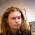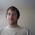Thursday, September 29, 2016

Give a second order statement true in (R,+) but false in (Q,+) or show there isn't one

Here is a logic question I will ask today and answer next week. Feel free to leave comments with
the answer- you may come up with a different proof than me and that would be great!

Our lang will have the usual logic symbols, quantification over the domain, quantification over subsets of the domain (so second order) the = sign, and the symbol +

Examples of sentences:

(∀ x)(∀ y)[ x+y=y+x]

true over Q,R,N.  False in S_n for n\ge 4 (group of perms of n elements)

(∃ x)(∀ y)[ x+y=y]

true in Q, R by taking 0. not true in {1,2,3,...}

Lets assume it is true and call the x 0

(∀ x)(∃ y)[x+y=0]

True in Q, R, Z, not true in N.

QUESTION ONE: Is there any sentence in the first order theory that is TRUE over (Q,+) but
FALSE over (R,+)?

QUESTION TWO: Is there any sentence in the second order theory that is TRUE over (Q,+)
but false over (R,+)?

1.How is {1,2,3,...} a nonabelian group?

1.The {1,2,3,...} refers to his second example, the nonabelian group thing was just a place where the first example sentence (which I believe has an extra "+y" in it) fails.

2.typo?
(forall x)(forall y)[ x+y=y+x+y]
shouldnt it be:
(forall x)(forall y)[ x+y=y+x]

3.You have an extra y+ in the first sentence

4.All typos fixed (I hope).
Thanks for corrections.

5.Bill, do you have a problem with using ∀ and ∃?!

1.I thought forall and exists written out were clear enough but better to use the real notation, so I have changed it. Thanks for motivating me to do so.

6.I think I have such a sentence. Rather than writing it out in LATeX I'm describing it in words. (Interesting how hard it was to come up with this - I study model theory but I so rarely think about second order quantification that it almost feels like magic.)

My sentence: you can write in the second order language, "there exists a binary relation P so that P satisfies all the finitely many first order axioms of a group-compatible linear order (think P(x,y) iff x, so they would behave as expected. Then the second sentence is true in R but not in Q.

So essentially we create < out of thin air and rely on the fact that there are no unexpected linear orderings possible on the divisible groups to show that in R, but not in Q, every bounded set has a least upper bound.

7.1) No (in particular, this follows from the Löwenheim–Skolem theorem).

2) Yes. Here is the sentence: For every x and y, there is z such that every subgroup (S, +) that contains z also contains x and y. This sentence can be written in the second-order language.

8.I think this works?

forall P Q, (forall a b, P(a) + P(b) = P(a + b) AND forall a b, Q(a) + Q(b) = Q(a + b) AND P(1) = Q(1)) IMPLIES forall a, P(a) = Q(a)

9.Ah, I'm not allowed that kind of quantification. Hmm...

10.There is a purely logical second order sentence P such that M |= P iff M is uncountable. The second-order theory of (R, +) contains P, but that of (Q, +) does not.

11.Quickly: the domain is uncountable

** Countability can be defined in SO with the + funciton in this way:

forall P . (P(0) and (P(x)->P(x+1))) // grab at least one infinite countable set ...

=> exists F . // ... which is a bijection ...
(forall x,y,z . F(x,y) and F(x,z) => y=z) and
(forall x,y,z . F(x,y) and F(z,y) => x=z) and

// ... from the domain to the countable set ...
(forall x exists y . F(x,y)) and
(forall x,y . F(x,y) -> P(y))

The negation of the above is in the SO of (R,+) but not in the SO of (Q,+)

12.... in the above "1" is not a member of the signature, but we can replace it with any non-zero element:
forall P . (exists d . ((!d=0) and P(0) and (P(x)->P(x+d)))) => ...

Another alternative simpler approach could be to use irrationals: i.e. define x^2 and use:

forall x,y . exists z . z^2 = x^2 + y^2

13.In the rationals there is a bounded set which doesn't have a least upper bound. This should be expressible in second-order logic.

14.The first-order theory of addition over rational numbers is complete so the answer is negative.

15.For second-order you can express the distance using addition and then express the sentence that a Cauchy sequence has a limit.

16.Even easier: Any two non-trivial additive groups in Q have a non-trivial intersection. Not true in R.